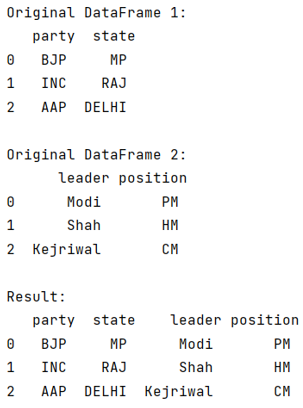# Combine two pandas dataframes with the same index

Given two pandas dataframes, we have to combine them with the same index.
Submitted by Pranit Sharma, on September 12, 2022

Pandas is a special tool that allows us to perform complex manipulations of data effectively and efficiently. Inside pandas, we mostly deal with a dataset in the form of DataFrame. DataFrames are 2-dimensional data structures in pandas. DataFrames consist of rows, columns, and data.

## Combining two pandas dataframes with the same index

We are given two DataFrames with the same index but different columns, we need to combine the two DataFrames with the same index but all the columns.

We will use pandas.concat() method for this purpose. The pandas.concat() is a method of combining or joining two DataFrames. It is used to combine DataFrames but it is a method that appends or inserts one (or more) DataFrame below the other.

Let us understand with the help of an example,

## Python code to combine two pandas dataframes with the same index

```# Importing pandas package
import pandas as pd

# Creating dictionaries
d1 = {
'party':['BJP','INC','AAP'],
'state':['MP','RAJ','DELHI']
}

d2 = {
'position':['PM','HM','CM']
}

# Creating DataFrame
df = pd.DataFrame(d1)
df2 = pd.DataFrame(d2)

# Display the DataFrame
print("Original DataFrame 1:\n",df,"\n")
print("Original DataFrame 2:\n",df2,"\n")

# Combining two DataFrames
res = pd.concat([df,df2],axis=1)

# Display result
print("Result:\n",res,"\n")
```

Output:What's New (MCQs)

Top Interview Coding Problems/Challenges!

Languages: » C » C++ » C++ STL » Java » Data Structure » C#.Net » Android » Kotlin » SQL
Web Technologies: » PHP » Python » JavaScript » CSS » Ajax » Node.js » Web programming/HTML
Solved programs: » C » C++ » DS » Java » C#
Aptitude que. & ans.: » C » C++ » Java » DBMS
Interview que. & ans.: » C » Embedded C » Java » SEO » HR
CS Subjects: » CS Basics » O.S. » Networks » DBMS » Embedded Systems » Cloud Computing
» Machine learning » CS Organizations » Linux » DOS
More: » Articles » Puzzles » News/Updates

© https://www.includehelp.com some rights reserved.